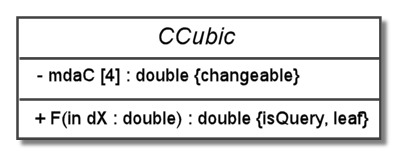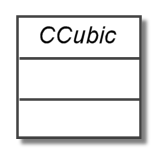# Bits & Bytes

## UML Class Diagrams – part 5

Continued . . .

For this section, we pull together the material for modeling operations and attributes to give a specification of the full form and then discuss modeling for the appropriate level of detail. For attributes and operations, the name is always used, but the rest of the elements are optional. By convention, the class name is in the top box, followed by attributes and operations, respectively. This is the common convention that we have used and it is highly recommended, but UML does allow for any ordering.

For attributes, we have full form shown below. The name is mandatory, and the rest of the optional elements are shown in C++ template-style brackets ( < > ) to indicate that they are optional. First, we have the visibility, which is shown as +, #, , or ~ for public, protected, private, or package level visibility, respectively. Then we have the name. The attribute could contain multiple instances of its type; the allowed range of multiplicity can be specified in bracket notation like this [0..1], [n], or [0..*], which correspond to {0, 1}, {n}, or {0, 1, 2, … } in set notation, respectively. After this, we have a colon followed by the type. Then an “=” and an initial value. Finally, we have a the properties list, each of which may have a value setting.

### Attributes

`<visibility> name <Multiplicity> <: Type> <= InitialValue> <{Properties}> `
###### Properties
`Property1 <= Value1>, ... `

For operations, the full form is shown below. Again, the optional elements are show in C++ template-style brackets. Just as with attributes, we have the visibility followed by the name. Inside the parentheses, we have the arguments, which can have a direction, name, type, and default value, as shown under the Arguments heading. After the arguments, we have a colon and the return type. Finally, we have the properties in braces, just as with the attributes.

### Operations

```<Visibility> Name(Arguments) <: ReturnType> <{Properties}>
```
###### Arguments
`<Direction1> <ArgName1> <: ArgType1> <= DefaultValue1> ; ...`
###### Properties
`Property1 <= Value1> , ...`

Below, we have a class for creating cubic polynomials. These are polynomials of the form

F(x) = c0x0 + c1x1 + c2x2 + c3x3

= c0 + c1x + c2x2 + c3x3,

where ci is a constant coefficient of the appropriate degree. The function F() is used to evaluate the polynomial at a particular value of x. For this class, we will assume that a constructor exists (not shown) that initializes the cubic to be the zero polynomial (all zero coefficients).

```class CCubic
{
public:
double F(double dX = 0.0) const;
private:
double mdaC;
};```

This class can be diagramed in UML, as shown below, with in the fullest form for attributes and operations. Here, we use the assumption that the cubic is initialized in a constructor somewhere to be the zero polynomial; of course, that make the polynomial a constant and not a cubic. The coefficients can be changed, so we indicate that with the “changeable” property. Also, the function F() is “const” because does not change the values of the coefficients. Moreover, it is statically bound. Therefore, we have modeled it with the property specifications “isQuery” and “leaf” to indicate these properties.This an elaborate specification, but is reasonable for modeling a simple class like this. However, for a diagram with many attributes and operations, this level of detail can get messy and difficult to read. Moveover, many of the details may not be relevant in a given context. For example, when designing this class it is probably not very important what the initial values of the coefficients are or the default value of dX in the function F(). So, we might use the cleaner version below.Likewise, the fact that the coefficients are “changeable” is probably obvious as are the properties of the function F(). Furthermore, the use of doubles is probably clear and may not even be material to the design. So, we can throw those out as well as the obvious fact that dX is an input parameter. Our greatly simplified diagram now looks like this.Finally, in a larger context, we may only be concerned that a cubic class exists and we can throw out the attributes and operations entirely, as we demostrate below. Notice that our diagram has three compartments, even though two ar empty. In this case, we could use simply one compartment with the class name or we could use three like this to indicate that it is important to keep in mind that this class has attributes and operations.By: Michael Hall